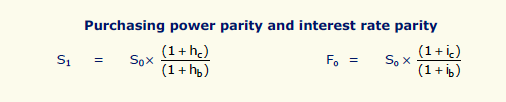AFMP4
Syllabus E. Treasury And Advanced Risk Management Techniques E2. The use of financial derivatives to hedge against forex risk

# E2b. Predicting Exchange Rates 2 / 13

### Syllabus E2b)

b) Evaluate, for a given hedging requirement, which of the following is the most appropriate strategy, given the nature of the underlying position and the risk exposure:

i) The use of the forward exchange market and the creation of a money market hedge

### Purchasing Power Parity (PPP theory)

Why do exchange rates fluctuate?

“The law of one price”#### Illustration

Item costs \$1,000
\$2:€ (base)
However inflation in US is 5% and Europe is 3%

According to law of one price what is the predicted exchange rate in 1 year?

• Solution

So next year - Item in US costs \$1,050 and in Europe €515
“The law of one price” = \$1,050 = €515

So, forward exchange rate = 1,050 / 515 = \$2.039:€1

PPPT “High inflation leads to depreciation of currency”

• Another way of calculating this is as follows:

Exchange rate now (counter) x (1+ Inf (counter) / 1 + inf (base))

2 x 1.05 / 1.03 = 2.039

#### Limitations

1. Future inflation is an estimate

2. Market is ruled by speculative not trade transactions

3. Governments often intervene

### Interest Rate Parity (IRP theory)

Why do exchange rates fluctuate?
An investor will get the same amount of money back no matter where he deposits his money

#### Illustration

US Interest rate = 10%
European Interest rate = 8%
Exchange rate = \$2:€

Investor has \$1,000 to invest for 1 year

What is the future exchange rate as predicted by IRPT?

• Solution

In US he will receive \$1,100 in one years time
In Europe he will receive €540
Forward rate will therefore be 1,100 / 540 = \$2.037:€

IRPT “High interest rates leads to depreciation of currency”

• Another way of calculating this is as follows:

Exchange rate now x (1+ Int (counter) / 1 + int (base))

2 x 1.10 / 1.08 = 2.037

#### Limitations

1. Government intervention